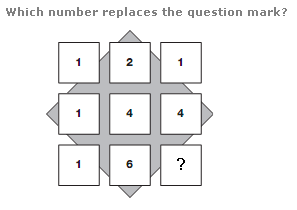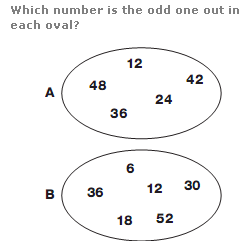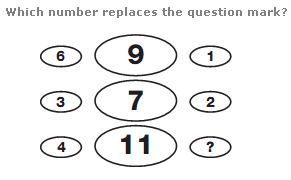# Puzzles - Number puzzles

### Exercise :: Number puzzlesAnswer : 9 Explanation : Working in rows, top to bottom, reading each row as a 3 digit number, rows follow the sequence of Square Numbers from 11 to 13.Answer : A:42 B:52 Explanation : In the first oval, all numbers are multiples of 4. In the second oval, all numbers are multiples of 3.Answer : 5 Explanation : In each row, add together the left and right hand numbers, then add 2 to give the central value.